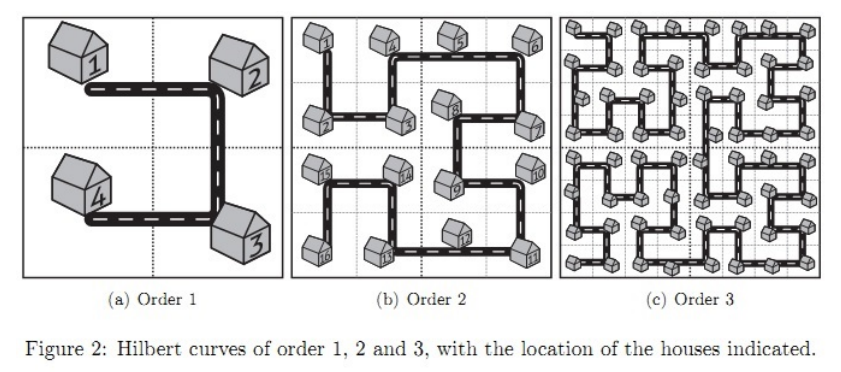奇怪的道路 (FCS2017 D2T1)

emmm… FCS2017 夏令营的一道题目……用的是分治的算法，为了题目的一些细节本蒟蒻足足推了两个小时QAQ……所以要写篇文章记录一下不然哪天自己就看不明白了……

奇怪的道路

问题描述样例输入

2 4 2 1 3 1 3 2 2 2 2 3 3 3 3 4 2 4

13 11 1 3

数据规模

Easy：对于30%的数据，1 ≤ 𝑛 ≤ 3。 Normal：对于60%的数据，1 ≤ 𝑛 ≤ 8。 Hard：对于100%的数据，均有1 ≤ 𝑛 ≤ 15，1 ≤ 𝑖1, 𝑗1, 𝑖2, 𝑗2 ≤ 2𝑛，1 ≤ 𝑇 ≤ 10000。

思路

1     2
=========
1 1 --- 2
|
|
|
2 4 --- 3

1     4
|     |
|     |
2 --- 3

// 右上
index = 1 * nodeNum + getnum(n - 1, x, y - edgeMid);

// 右下
index = 2 * nodeNum + getnum(n - 1, x - edgeMid, y - edgeMid);

// 左上
index = getnum(n - 1, y, x);

// 左下
index = 3 * nodeNum + getnum(n - 1, edgeMid + 1 - y, 2 * edgeMid + 1 - x);

示例代码 (C++)

#include <bits/stdc++.h>
int getNum(int stage, int x, int y)
{
if (stage == 1) {
if (x == 1 && y == 1)
return 1;
if (x == 1 && y == 2)
return 2;
if (x == 2 && y == 2)
return 3;
if (x == 2 && y == 1)
return 4;
}

int edgeMid = 1 << (stage - 1),                   // edgeMid = 2^(stage-1)
nodeNum = 1 << (2 * (stage - 1));   // nodeNum = 4^(stage-1) = 2^2(stage-1)

if (x <= edgeMid && y <= edgeMid)
return getNum(stage - 1, y, x);
if (x <= edgeMid && y > edgeMid)
return nodeNum + getNum(stage - 1, x, y - edgeMid);
if (x > edgeMid && y > edgeMid)
return 2 * nodeNum + getNum(stage - 1, x - edgeMid, y - edgeMid);
if (x > edgeMid && y <= edgeMid)
return 3 * nodeNum + getNum(stage - 1, edgeMid + 1 - y, 2 * edgeMid + 1 - x);

}

int main()
{

int n, T;
scanf("%d%d", &n, &T);

while (T--)
{
int x1, y1, x2, y2;
scanf("%d%d%d%d", &x1, &y1, &x2, &y2);
printf("%d\n", abs(getNum(n, x1, y1) - getNum(n, x2, y2)));
}

fclose(stdin);
fclose(stdout);

return 0;
}

• 原题版权归出题人所有。©2016-2019  宇宙よりも遠い場所 / Published with Hugo / CC-BY-SA 4.0 Licensed